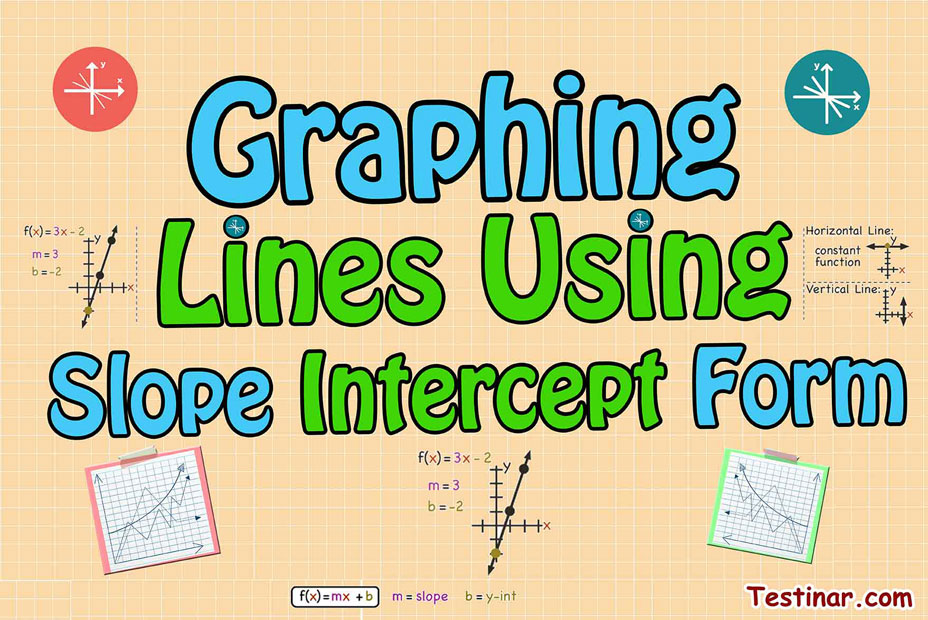## How to Graph Lines by Using Slope–Intercept Form?

There are several ways to graph linear equations. Utilizing slope-intercept form is one of the fastest and simplest methods of graphing a linear equation. Prior to starting, we have to explain some of the vocabulary. We will discuss $$x$$ and $$y$$ intercepts.
An $$x$$ intercept is the point where the line crosses the $$x$$-axis. The $$y$$ intercept is the point where the line crosses the $$y$$-axis.
We're merely going to concentrate on the $$y$$ intercept for this lesson, however, you will have to know about the $$x$$ intercept for later.

### Look over at intercepts.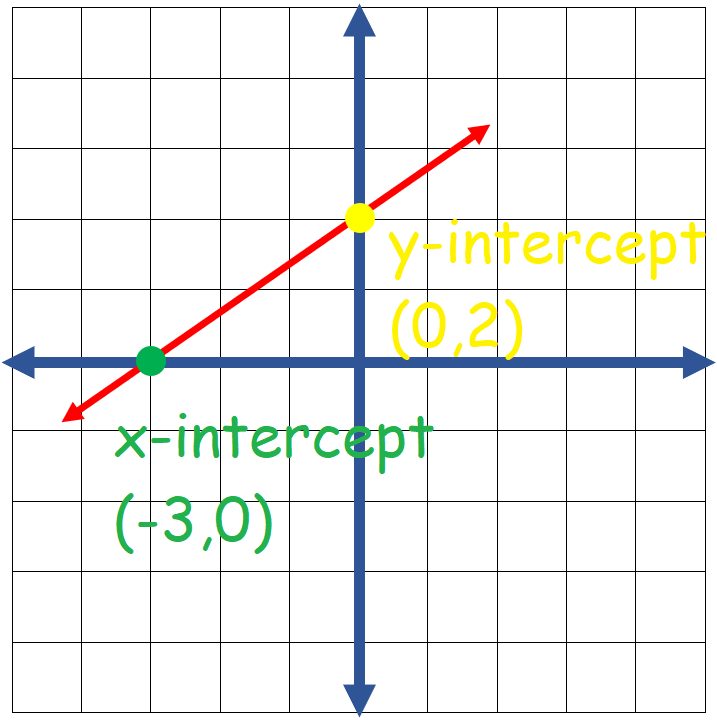Slope intercept form is utilized whenever the linear equation is written as: $$y \ = \ mx \ + \ b$$
$$x$$ and $$y$$ are the variables. $$m$$ is going to be a numeral, which is your slope. $$b$$ is also going to be a numeral and that is the $$y$$-intercept.

In this form only (whenever the equation is written as $$y \ = \ ...$$ ) the coefficient of $$x$$ is the slope and the constant is the $$y$$ intercept.
Whenever an equation is written in this form, you can view the equation and have sufficient info for graphing it.
For instance, imagine you have the equation $$y \ = \ 3x \ + \ 1$$ and are told to graph it.
Straight from the equation, you can see the $$y$$-intercept is $$1$$. Plus you know the slope is $$3$$.
$$slop \ = \ \frac{∆y}{∆x} \ = \ \frac{3}{1} \ = \ 3$$
Therefore, for every one unit you go to the right, so we have to go up three units: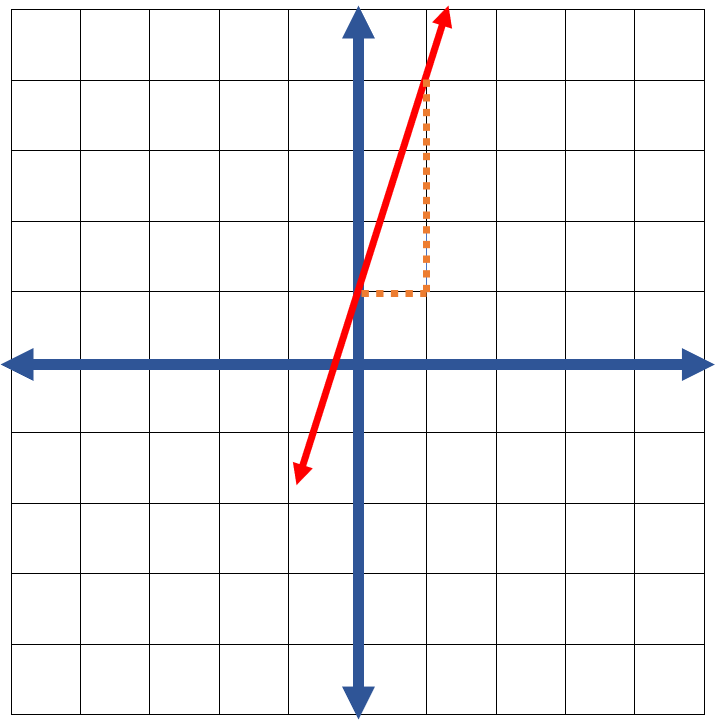A first quadrant coordinate plane. The $$x$$ and $$y$$-axes each scale by one. A graph of the line goes through the points $$(0,1)$$ and $$(1,4)$$ which have been plotted. There’s a horizontal segment from $$(0,1)$$ to $$(1,1)$$. There’s a vertical line from $$(1,1)$$ to $$(1,4)$$.

Here’s the final graph: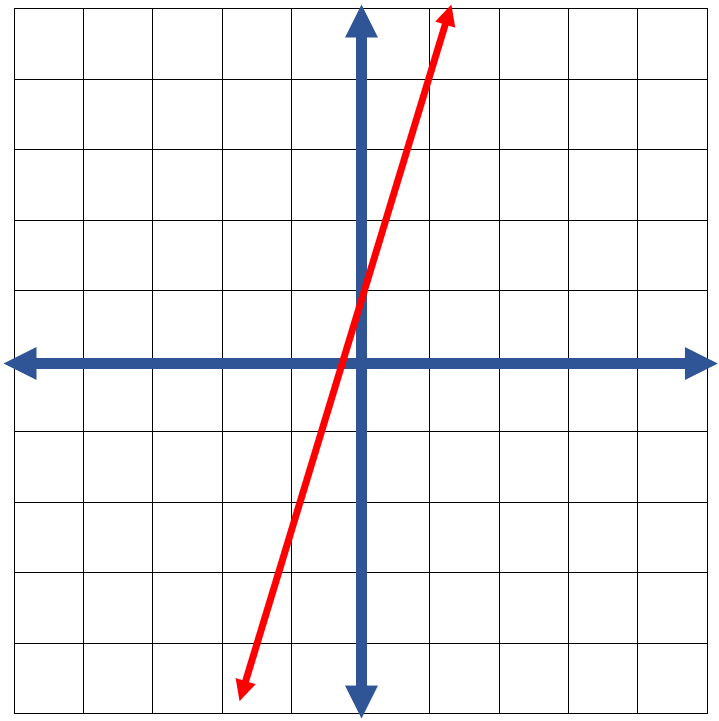### Exercises for Graphing Lines Using Slope Intercept Form

1) Sketch the graph of the line: $$y \ = \ 3x \ + \ 2$$

2) Sketch the graph of the line: $$y \ = \ 2x \ + \ 1$$

3) Sketch the graph of the line: $$y \ = \ x \ + \ 5$$

4) Sketch the graph of the line: $$y \ = \ 3x \ - \ 2$$

5) Sketch the graph of the line: $$y \ = \ x \ - \ 2$$

6) Sketch the graph of the line: $$y \ = \ 4x \ + \ 1$$

7) Sketch the graph of the line: $$y \ = \ 2x \ - \ 3$$

8) Sketch the graph of the line: $$y \ = \ 3x \ - \ 4$$

9) Sketch the graph of the line: $$y \ = \ \frac{1}{2} \ x \ + \ 1$$

10) Sketch the graph of the line: $$y \ = \ 2x \ - \ 5$$

1) Sketch the graph of the line: $$y \ = \ 3x \ + \ 2$$

$$\color{red}{m \ = \ 3, \ b \ = \ 2 \ ⇒}$$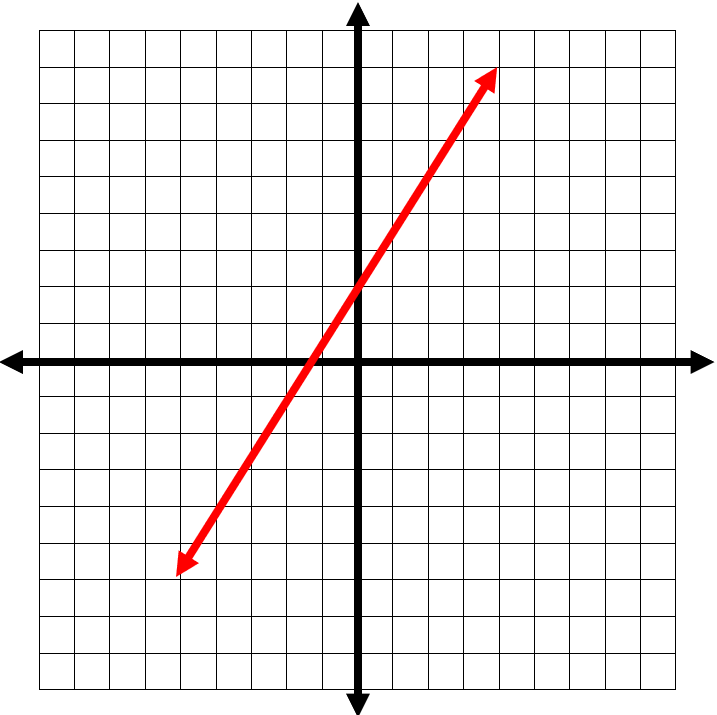2) Sketch the graph of the line: $$y \ = \ 2x \ + \ 1$$

$$\color{red}{m \ = \ 2, \ b \ = \ 1 \ ⇒}$$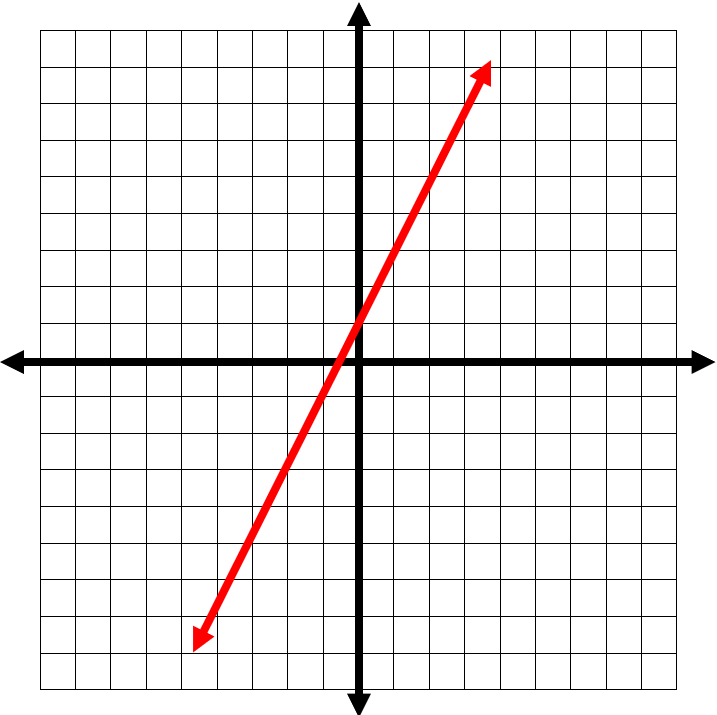3) Sketch the graph of the line: $$y \ = \ x \ + \ 5$$

$$\color{red}{m \ = \ 1, \ b \ = \ 5 \ ⇒}$$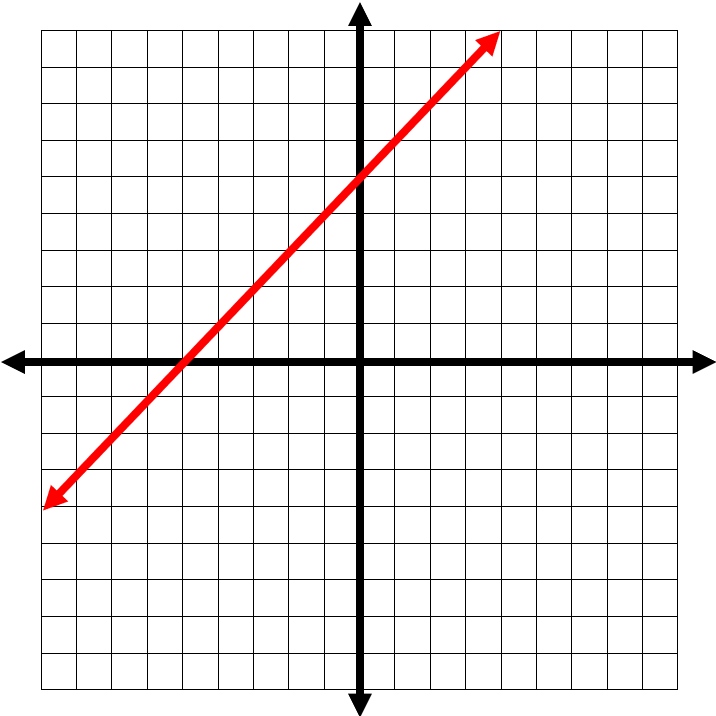4) Sketch the graph of the line: $$y \ = \ 3x \ - \ 2$$

$$\color{red}{m \ = \ 3, \ b \ = \ -2 \ ⇒}$$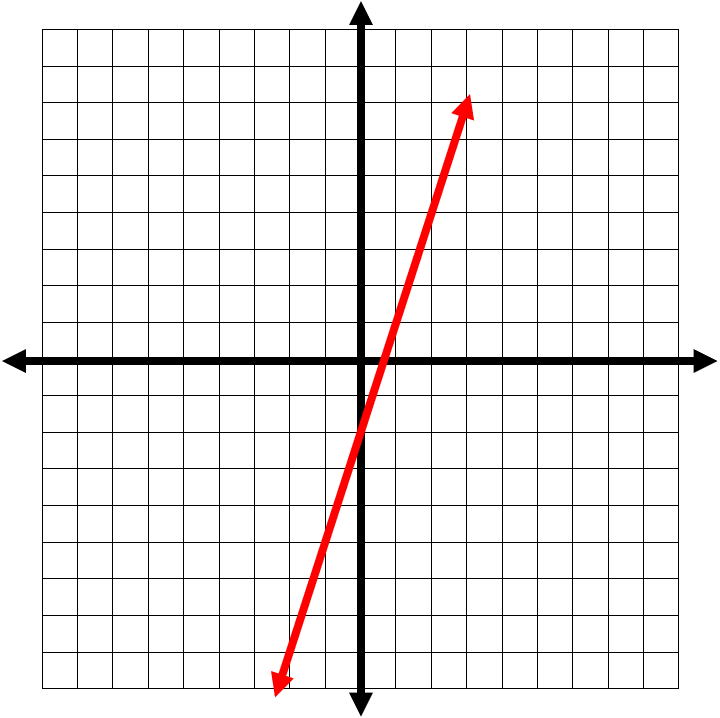5) Sketch the graph of the line: $$y \ = \ x \ - \ 2$$

$$\color{red}{m \ = \ 1, \ b \ = \ -2 \ ⇒}$$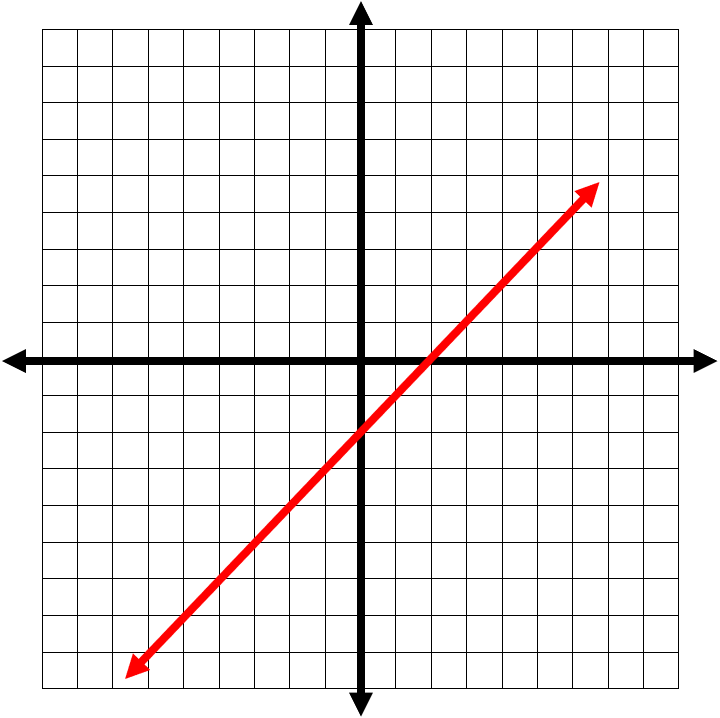6) Sketch the graph of the line: $$y \ = \ 4x \ + \ 1$$

$$\color{red}{m \ = \ 4, \ b \ = \ 1 \ ⇒}$$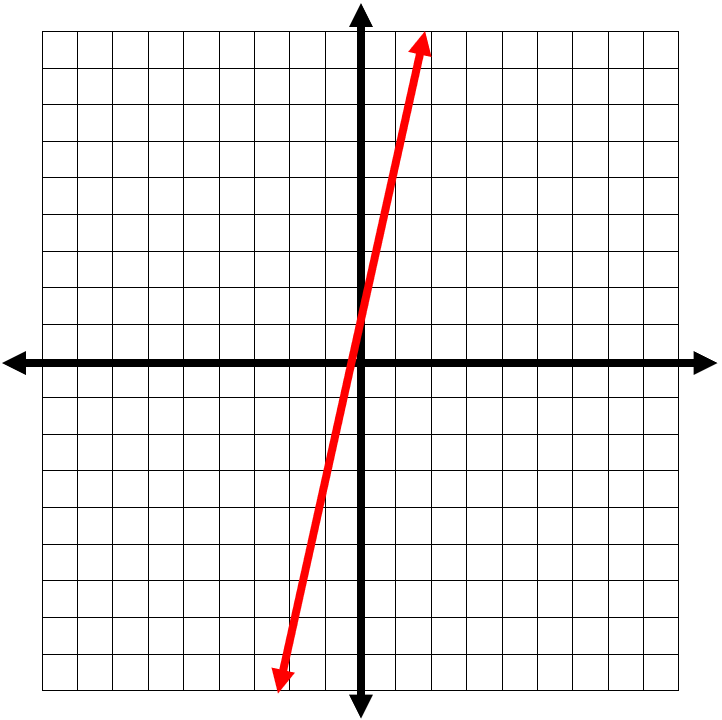7) Sketch the graph of the line: $$y \ = \ 2x \ - \ 3$$

$$\color{red}{m \ = \ 2, \ b \ = \ -3 \ ⇒}$$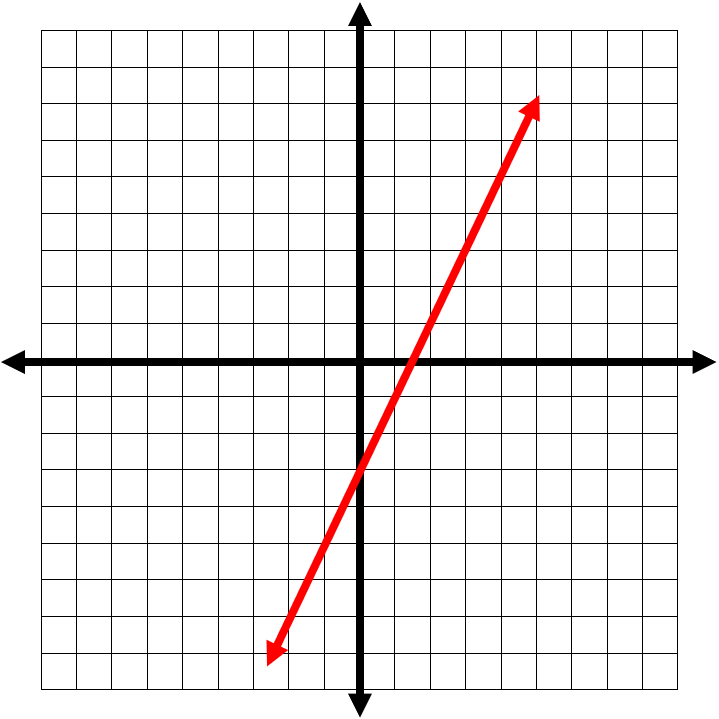8) Sketch the graph of the line: $$y \ = \ 3x \ - \ 4$$

$$\color{red}{m \ = \ 3, \ b \ = \ -4 \ ⇒}$$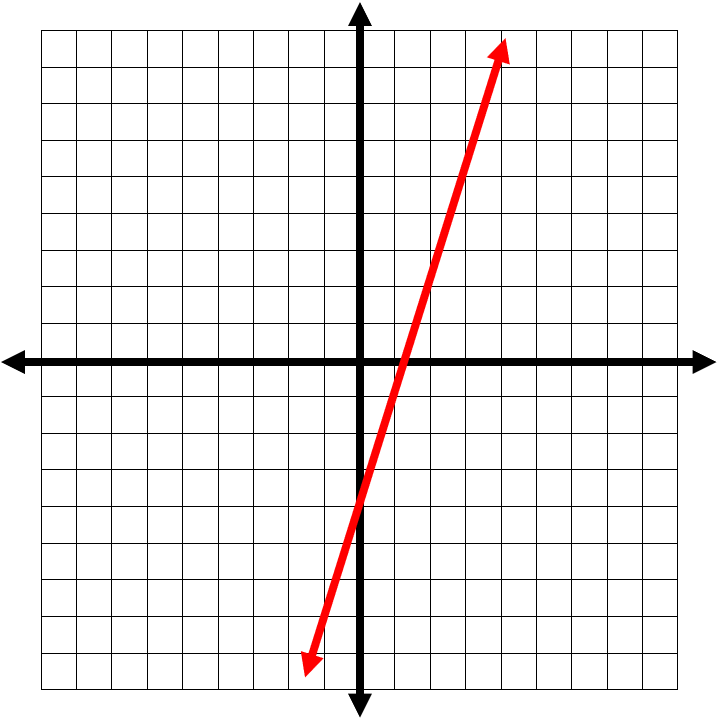9) Sketch the graph of the line: $$y \ = \ \frac{1}{2} \ x \ + \ 1$$

$$\color{red}{m \ = \ \frac{1}{2} \ , \ b \ = \ 1 \ ⇒}$$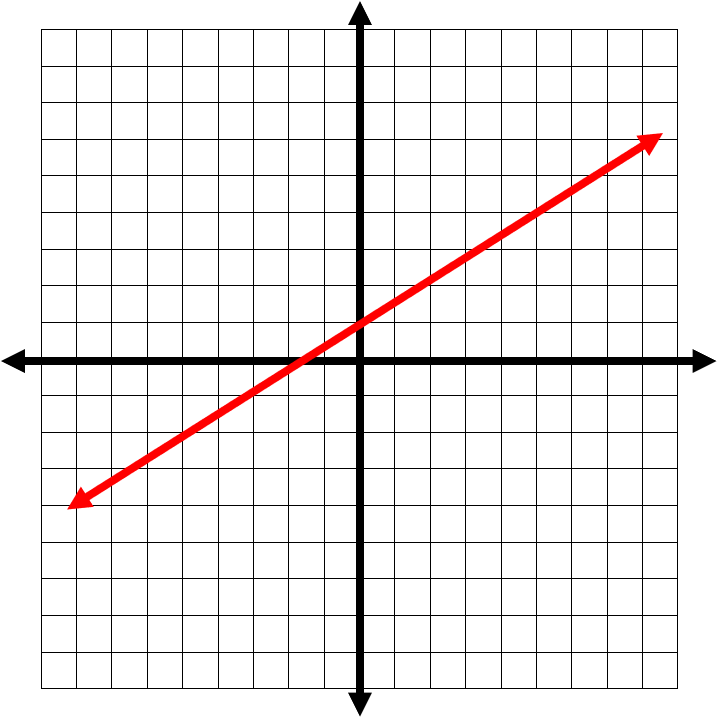10) Sketch the graph of the line: $$y \ = \ 2x \ - \ 5$$

$$\color{red}{m \ = \ 2, \ b \ = \ -5 \ ⇒}$$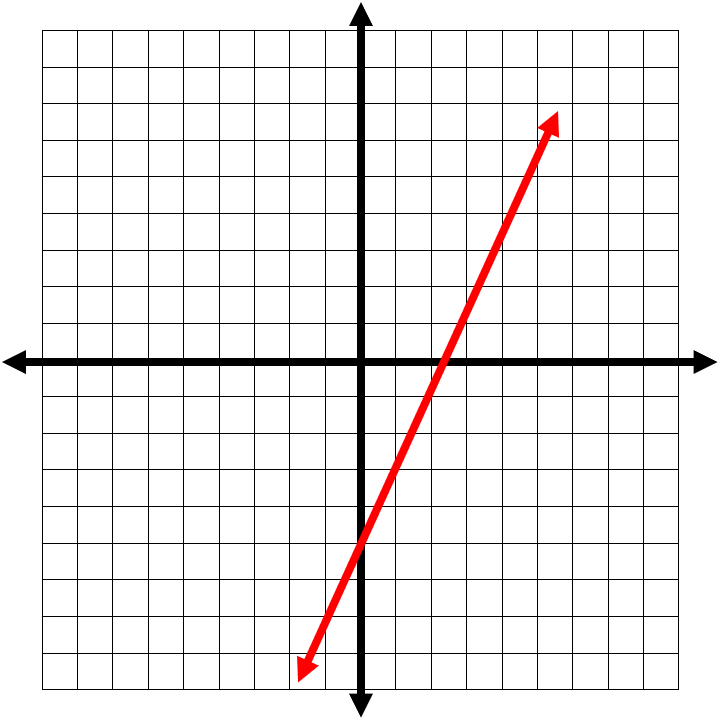## Graphing Lines Using Slope Intercept Form Quiz

### The Most Comprehensive Praxis Core Math Preparation Bundle

$76.99$36.99

### STAAR Grade 8 Math Workbook

$21.99$16.99

### Comprehensive DAT Math Practice BooK

$17.99$12.99

### SHSAT Math Practice Workbook

$25.99$14.99